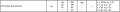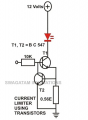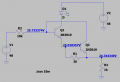# How to select the value of Base-Emitter resistor?.

#### pinkyponky

Joined Nov 28, 2019
270
Hi all,

While I'm searching for the answer for my question, I found the post thread by title named as "What is use of R41 (base to emitter) resistor in Transistor Relay Circuit ?".

But, one of the post in the thread stated that, first need to be calculated the leakage current of the transistor. Again, I don't know how to calculate the leakage current. However, MisterBill2 posted the text by saying what are the thinks need to be calculated to find out the leakage current.

The text from MisterBill2:
[
OK, the process for determining that resistor starts elsewhere. As already mentioned, first the required collector current to operate the relay with a worst case transistor saturation voltage must be known. That will require reading the specification sheet.
Next, the base current to bring the switching transistor into saturation, for a minimum gain transistor must be calculated.
Once the required base current is known, the value of R3, the base current limiting resistor can be selected, allowing for both the base-to emitter voltage to vary with temperature, and allowing some current through R41 (R4??). The value of R4 is then selected to pull the base of the transistor below the cutoff voltage even at the maximum leakage current of the opto-isolator plus the off-state base leakage of the transistor.
Of course an adequate margin must be used to allow for tolerance variations of the resistor values. Then an appropriate standard value for the resistor is selected. This is why most engineers just pick values based on experience and common practice.]

In my case, I don't have any relay in my circuit. Just I'm learning how to calculate the Rbe resistor?. I'm not understand that much from the text, but, I have tried my way please could you also help me.

1. Max. case Collector-Emitter Saturation Voltage (Vce) - 305mV (according to ZXTN25060BZ datasheet)
2. Max. Collector current (Ic) - 5A (according to ZXTN25060BZ datasheet)
3. Min. DC current gain (hFE) - 20 (according to ZXTN25060BZ datasheet)

I have just assumed and consider that 1A current (Ic) is the required and 5V (Vbe), such that I have calculated the Rb.

According to datasheet (from page 5), 50mA (Ib) current is required to turn on the transistor to pass 1A current from collector to emitter.

Rb = (Vbe - Vbe(on)) / Ib => (5-1.050) / 50m => 79 ohms ( according to datasheet Vbe(on) = 1.050V)

From here on-wards not understand what I have to do to calculate the leakage current of the transistor. Please could you help me?.

Thank you, all.

#### BobTPH

Joined Jun 5, 2013
4,899
I have never calculated a base to emitter resistor. If I use one at all, I use 10K. You are overthinking a non-problem.

Leakages cannot be calculated, look for it in the datasheet.

Bob

•ericgibbs

#### pinkyponky

Joined Nov 28, 2019
270
Dear Bob,

The leakage current is not given in the datasheet.

However, how to know the value of Rbe resistor?. As I knew that the Rbe is used to discharge the energy that is existing at the base terminal. I have seen lot of example circuits there are using different resistor values, so that's why I rise this question.

#### dl324

Joined Mar 30, 2015
14,458
how to know the value of Rbe resistor?
It isn't always required. Post a schematic so we can see what you're working with.

#### pinkyponky

Joined Nov 28, 2019
270
Hi Dennis,

How to know that when the Rbe resistor is required and when its not required?.

Just I'm learning the below article/image and just I have considered ZXTN25060BZ instead of BC547 transistor.

I have some questions after gone through the datasheet (ZXTN25060BZ) and the below article, please, anyone could you explain clearly.

1. I understand that the current will amplify with the darlington configuration, by following the below formulas:
1. Ic = β*Ib => β = β1* β2 + β1 + β2
Ie = Ib+Ic
2. Here, My question is; How to read and select the β value (from my choosen parameters such as Vb=48V, Ib=4.8mA, and Vc=12V) from the datasheet?, Since the couple of β values are listed in the datasheet (ZXTN25060BZ) as shown below.3. For example, If Vce = 12V (from the below circuit), then there is no such condition to choose the gain. In this case, how to chose the gain value to calculate the output current of the darlington amplifier.
4. If I know how to read the β value from the datasheet, then I think I understand the darlington configuration used for current amplifying purpose.
2. As stated in the below article/image, how the current is limiting by using the same configuration as a darlington?. How the Rbe resistor will involvi in this functionality (as a current limiting functionality)?.
3. Why 560m ohms resistor used in the below circuits?. Is there any reason?. How they choose that?.

I hope you recognizing my work. Please anyone could you help me?.

https://www.brighthubengineering.com/diy-electronics-devices/66646-basic-transistor-circuits-for-beginners-explored/#:~:text=Current Amplifier: Using just a,amplified by the second transistor.Last edited:

#### pinkyponky

Joined Nov 28, 2019
270
Dear Ericgibbs,

Please could you also answer me the question which I have asked regarding the β in the above post?.

Thank you, Eric!.

#### pinkyponky

Joined Nov 28, 2019
270
Hi Eric,

Please could you explain me, how the base voltage is 1.347V?.

#### MrChips

Joined Oct 2, 2009
26,089
For each transistor to be in the active region Vbe must be between 0.6 and 0.7V.
Vbe for both Q1 and Q2 add up to 1.2-1.4V.
The current through Q1 is about 0.6V/30Ω = 20mA.

•pinkyponky

#### pinkyponky

Joined Nov 28, 2019
270
Hi MrChips,

Just for fun I have added load as a 1k to understand the functionality of the circuit.

I have change the transistors because maximum Vce voltage limitations.

I have added three voltage values in the circuit. I know that there will be voltage drop 0.6V - 0.7V between each voltages. But, Still I'm not understand how the base voltage of Q1 is 25.733V.

Please could you explain this as well.

Thank you, MrChips.#### BobTPH

Joined Jun 5, 2013
4,899
The voltage at the emitter is about 25, the voltage at the base is about 25.7, so Vbe is about 0.7V.

Bob

#### MrChips

Joined Oct 2, 2009
26,089
In the previous circuit Q2 collector current does not flow through R1.
In the new circuit Q2 collector current flows through R3.
Now, the current through R3 is 20mA + 4.3mA = 24.3mA.

The voltage at the base of Q1 is 24.3V + 1.4V = 25.7V.

#### dl324

Joined Mar 30, 2015
14,458
How to know that when the Rbe resistor is required and when its not required?
Only an issue with saturation mode operation. The attached lecture from Columbia University seems believable. Phenomenon discussed on page 3. The discussion of turn-off time starts on page 7. I'd just start with 10k and adjust as necessary...

EDIT: This might also be helpful: Schottky transistor - Wikipedia

#### Attachments

• 144.3 KB Views: 1
Last edited:

#### pinkyponky

Joined Nov 28, 2019
270
The voltage at the emitter is about 25, the voltage at the base is about 25.7, so Vbe is about 0.7V.

Bob
Hi Bob,

How 25V present at Q1_Emitter?. Please don't tell that Vbe (Q1, Q2) about 0.7V, this functionality I have understand. How originally this voltage?.

#### DickCappels

Joined Aug 21, 2008
8,707
Are you taking into account that Vbe is the voltage across the base-emitter junction? It is not necessarily any particular value relative to ground.

In circuit design Vbe is one of the things you can truly count on even without a datasheet. It only varies considerably by semiconductor material.

#### BobTPH

Joined Jun 5, 2013
4,899
What do you think the voltage at Q1’s emitter should be and why?

Bob

#### ericgibbs

Joined Jan 29, 2010
15,517
Hi pp,
You already know that Q1 and Q2 are a nominal ~20mA current circuit, also you also have a 48Vdc drive signal via a 10k, [4.8mA] so why are you unable to calculate the voltage drop across the 1k resistor.????

Do you know Ohms law equations.???

E

#### BobTPH

Joined Jun 5, 2013
4,899
I am hoping an answer to my question will tell us what he is getting wrong.

Bob